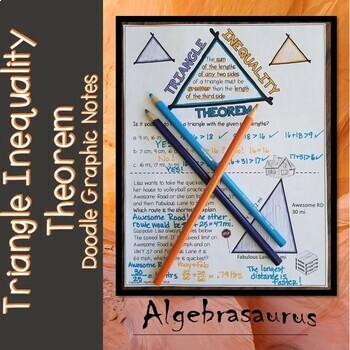DID YOU KNOW:
Seamlessly assign resources as digital activities

Learn how in 5 minutes with a tutorial resource. Try it Now

Learn More# Triangle Inequality Theorem Doodle Graphic Organizer6th - 11th
Subjects
Resource Type
Formats Included
• PDF
• Compatible withActivities
Pages
4 pages
Compatible with Easel Activities
Create an interactive version of this PDF students can complete on any device. Easel is free to use! Learn more.

### Description

Doodle graphic organizer used to develop an understanding of the Triangle Inequality Theorem. Allow your students the ability to use visual cues to aid in memory and recall. The document prints on standard paper but can be trimmed to fit a composition notebook (that's how I use it). A key is included.

I have used math journals in my classroom for almost 20 years. I implemented interactive journals and struggled with the cut and paste and time involved in their creation. While I know there is a benefit, it did not work for me. These notes are the answer for my teaching style. While students are required to fill in vital information, they are not required to color. My students love getting an interesting handout instead of a generic math worksheet.

OTHER PRODUCTS YOU MIGHT ENJOY:

BEST DEAL!

ALGEBRA

Distance & Midpoint Formulas

Rational Exponent Form vs. Radical Form

Arithmetic Sequences & Series

Geometric Sequences & Series

Factoring Flow Chart

Solving Absolute Value Equations

Systems of Equations in 2 Variables

Solving Systems of Equations in 3 Variables

Cramer’s Rule

Sets of Real Numbers

Evaluating Determinants

Operations on Functions

Inverse Functions

Polynomial Division: Long & Synthetic

Descartes Rule of Signs

Logarithms and their Properties

Natural Logarithms and e

Complex Conjugates Theorem

Possible Rational Zero Theorem

Referene & Coterminal Angles

Rational Expressions & Equations

GRAPHING

Square Root Functions

Standard Form of a Linear Equation

Graphing Piecewise Functions

Graphing Absolute Value Functions

Graphing Circles using Standard Form

Ellipses: Graphing & Equations

GEOMETRY

Points, Lines, & Planes Packet

Angles & Parallel Lines and Transversals

Triangle Circumcenter, Incenter, Centroid Theorems

Triangle Angle-Sum & Exterior Angle Theorems

Isosceles & Equilateral Triangles

Triangle Congruence: CPCTC, SSS, SAS, AAS, ASA

Perpendicular Bisector & Angle Bisector Theorems

Triangle Inequality Theorem

Polygon Interior Angle Sum & Exterior Angle Sum Theorems

Parallelogram Theorems & Tests for Parallelograms

Rectangle Theorems

Rhombi & Squares

Trapezoid Theorems

Kite Theorems

Similar Polygons

Similar Triangles

Geometric Mean & Triangle Altitudes

Special Right Triangles

Circle Terminology

Circle Central Angles & Arc Measure

Circle Arc Length

Circle Arcs & Chord Theorems

Circle Inscribed Angle Theorems

Tangets to a Circle

Secant & Tangent Theorems

Special Segments in a Circle Theorems

TRIGONOMETRY

Right Triangle Trig SOH CAH TOA

Finding Exact Trig Values

Trig Functions DEGREES Packet: Graphing & Equations

Trig Functions RADIANS Packet: Graphing & Equations

Units

Geometry Unit: Points, Lines, & Planes

Geometry Unit: Circles

Total Pages
4 pages
Included
Teaching Duration
N/A
Report this Resource to TpT
Reported resources will be reviewed by our team. Report this resource to let us know if this resource violates TpT’s content guidelines.